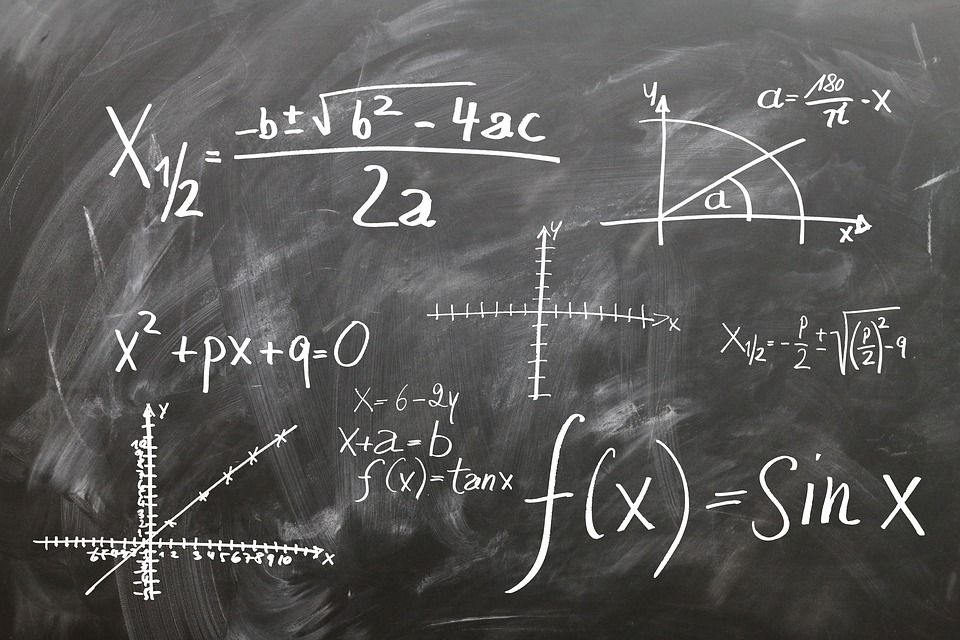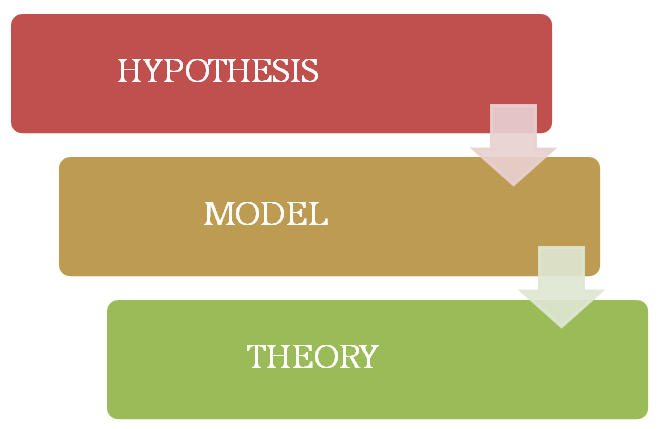# How are Models Related to Theories and Hypotheses

Theories and hypothesis are an integral part of models, which reflect real world phenomena. Thus, this article intends to explain,

1. What is a Hypothesis
2. What is a Theory
3. What is a Model
4. How are Models Related to Theories and Hypotheses

## What is a Hypothesis

A hypothesis is “a tentative explanation for an observation, phenomenon, or scientific problem that can be tested by further investigation” (American Heritage dictionary). It can be also explained as an educated guess that is based on observation and prior knowledge. This guess must go through a number of tests to prove or disprove something. A hypothesis becomes a theory once it is tested.

## What is a Theory

Theory can be defined as a “set of statements or principles devised to explain a group of facts or phenomena, especially one that has been repeatedly tested or is widely accepted and can be used to make predictions about natural phenomena” (American Heritage dictionary). A theory is a principle or a set of principles that scientifically explains a phenomenon. Such an explanation only becomes a theory, once a hypothesis is repeatedly tested and proven with sufficient evidence. Theories may also be disproven after rigorous testing when a new theory contradicts the old one.

### Difference Between Hypothesis and Theory## What is a Model

A scientific model is somewhere between a hypothesis and a theory. A hypothesis becomes a model when some testing has been done and the hypothesis becomes a valid observation. A theory is a model that has been repeatedly tested and proven.

Scientists often use scientific models to explain real world phenomenon by comparing them. Testability is one of the main requirements of a scientific model; they are only accepted by scientists once they have been tested in the real world.

Some examples of scientific models:

Plate tectonic model – the outer layer of earth as broken into segments called plates, which slowly drift around.

Biological evolutionary model – explains how the existing species are originated from the earlier species through the process of natural selection.

Spherical model – explains the spherical shape of the earth.

### Difference Between Model and Theory

Let’s now see how models are related to theories.

## How are Models Related to Theories and Hypotheses

Hypothesis and theories are an integral part of models. Hypotheses serve as the foundation of scientific models. Once a testable hypothesis has been tested several times and is confirmed as a valid observation, it is accepted as a model. A model that successfully explains a phenomenon can become a part of a theory. Once a model is repeatedly tested and confirmed it becomes an accepted theory. This is the relationship between theory, hypothesis, and models.Models must always be based on solid, scientific principles. A model elucidates a phenomenon by explaining how the said phenomenon occurs as a result of another phenomenon. They can be also used to make reliable predictions about some phenomena. Thus, models are very valuable in order to explain a phenonmenon.

### Summary

• A hypothesis is an educated, testable guess based on prior knowledge and observation.
• A hypothesis becomes a model after it is tested several times and is accepted as a valid observation.
• A model becomes a theory or a part of a theory once it is repeatedly tested and confirmed.

Image Courtesy: Pixabay